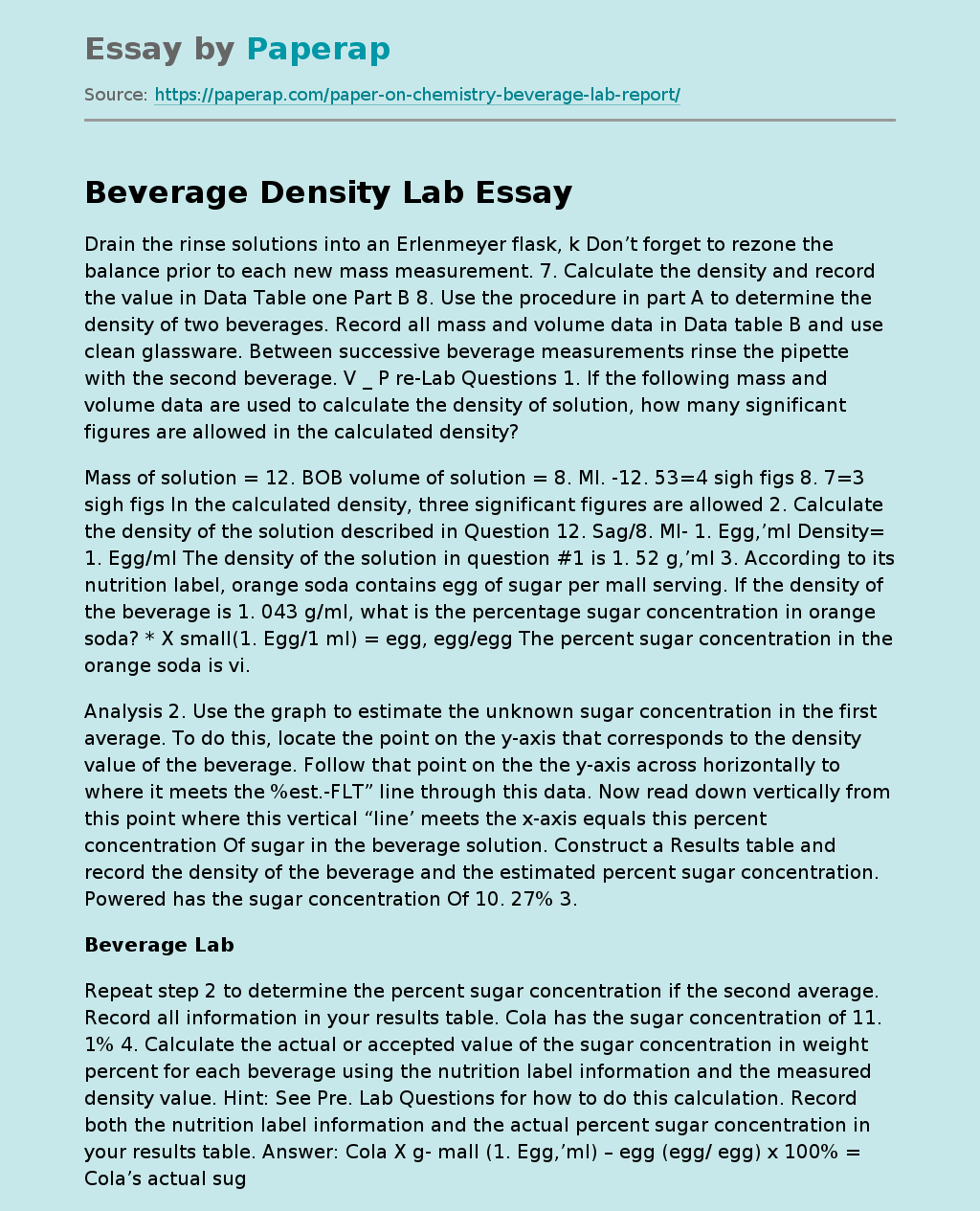# Beverage Density Lab

Drain the rinse solutions into an Erlenmeyer flask, k Don’t forget to rezone the balance prior to each new mass measurement. 7. Calculate the density and record the value in Data Table one Part B 8. Use the procedure in part A to determine the density of two beverages. Record all mass and volume data in Data table B and use clean glassware. Between successive beverage measurements rinse the pipette with the second beverage. V _ P re-Lab Questions 1. If the following mass and volume data are used to calculate the density of solution, how many significant figures are allowed in the calculated density?

Mass of solution = 12.

BOB volume of solution = 8. Ml. -12. 53=4 sigh figs 8. 7=3 sigh figs In the calculated density, three significant figures are allowed 2. Calculate the density of the solution described in Question 12. Sag/8. Ml- 1. Egg,’ml Density= 1. Egg/ml The density of the solution in question #1 is 1. 52 g,’ml 3. According to its nutrition label, orange soda contains egg of sugar per mall serving.

If the density of the beverage is 1. 043 g/ml, what is the percentage sugar concentration in orange soda? * X small(1. Egg/1 ml) = egg, egg/egg The percent sugar concentration in the orange soda is vi.

Analysis 2. Use the graph to estimate the unknown sugar concentration in the first average. To do this, locate the point on the y-axis that corresponds to the density value of the beverage. Follow that point on the the y-axis across horizontally to where it meets the %est.-FLT” line through this data.

Get quality help nowMarrie pro writerVerified

Proficient in: Density5 (204)

“ She followed all my directions. It was really easy to contact her and respond very fast as well. ”+84 relevant experts are online

Now read down vertically from this point where this vertical “line’ meets the x-axis equals this percent concentration Of sugar in the beverage solution. Construct a Results table and record the density of the beverage and the estimated percent sugar concentration. Powered has the sugar concentration Of 10. 27% 3.

## Beverage Lab

Repeat step 2 to determine the percent sugar concentration if the second average. Record all information in your results table. Cola has the sugar concentration of 11. 1% 4. Calculate the actual or accepted value of the sugar concentration in weight percent for each beverage using the nutrition label information and the measured density value. Hint: See Pre. Lab Questions for how to do this calculation. Record both the nutrition label information and the actual percent sugar concentration in your results table. Answer: Cola X g- mall (1. Egg,’ml) – egg (egg/ egg) x 100% = Cola’s actual sugar concentration is 11% overhead X mall (1. Egg / mall (15/240) x 100% 6. 25% Parade’s actual sugar concentration is 6. 25% S. Use the following equation to calculate the percent error in your experimental determination of the sugar content each beverage. Enter the percent error in the results table. Powered % Error = 18. 3-6. 1 / 8. 31* 100% = 22% The percent error for Powered is 22% % Error 11. 1 -11 The percent error for Cola is 0. 9% 6. What was your measured density for pure water (0% sugar solution)? The density of water is usually quoted as 1. 00 g,’ml, but this precise value is for C. Comment on why your measured density might be higher or lower than 1. 0 g/ ml. The measured density for pure water got 1. Egg/ ml_, because my water was exactly C. 7. This lab looks at the relationship between the density of a beverage and its sugar content. What assumption is made concerning the other ingredients in the beverage and their effect on the density of the solution? Do you think this is a valid assumption? Explain. Despite this lab looks just at the relationship between the density tot a beverage and its sugar content, other ingredients are also shown in the solution, like sodium. These other ingredients throw off the estimated percentage since they make the solution denser. . When plotting the data such as that obtained in this experiment, why is it not appropriate to “connect the dots”? It you were to repeat the lab, do you think you would get the exact same result? Comment of the sources of error in this experiment and their likely effect on the results. 1) It is not appropriate to “connect the dots” when you plot the data such as that in this experiment. It is because the measurements are imperfect. This means that the dots are not in a straight line, but a best fit lie helps approximate reality by using a straight line. 2) The results would not be exact, if were doing the lab again.

It probably would e more accurate, since I know the where I made errors. Would also consider about the unconsidered ingredients like sodium to get more accurate result. Is Conclusion Through the Beverage Density Lab, learned that the density of any material is determined by measuring its mass and volume and then dividing the mass by the volume. The mass of a substance can be measured directly using a balance for accuracy. I also learned how unconsidered ingredients, cleaning lab materials are a crucial part of the lab that can affect the results of the experiments. Now I know how to reduce my errors and get a more accurate result.